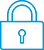cancel
Showing results for
Search instead for
Did you mean:It has been a while since anyone has replied. Simply ask a new question if you would like to start the discussion again.Level 1
5 2 0 1
Message 1 of 6
968
Flag Post

Solved!

# HP 10bII+ Issue when adding percentagesHP Recommended
10bII+

am studying accounting and finance and just bought this calculator which I'm still trying to figure out how to use. I was trying to work on a formula for required rate of return R=Rf+(Rm-Rf)*B with the following values

R=4%+(8%-4%)*1.5

When I do this on excel the answer I get is 10%, however the calculator does not give me the same answer. My initial inputs where as follows:

4%+(8%-4%)*1.5 = 0.16 or 15.52%  As I was doing the calculation I notices something

4% transforms into 0.040

+

(

8% transforms into 0.080

-

4% transforms into 0.0032

)

After closing parenthesis I get 0.077 which I assume is the sum of 0.040 and the result from the operation within the parenthesis.

* 1.5

= 0.16

I have tried to do this a couple different ways and doing each step separetly, but I always come to the same issue when doing the part inside the parenthesis. What am I doing wrong?

I've had the calculator for about 2 days and honestly am having a bit of a hard time working it out. Any help is appreciated.

Thanks.

Tags (1)
5 REPLIES 5Level 9
5,904 5,889 206 526
Message 2 of 6
Flag PostHP Recommended

Hi

I am confused also.

4% + (8% - %4) * 1.5  in a spreadsheet gives 0.1 or 10%

=SUM(0.04 + (0.08 - 0.04) *1.5)  0.1

=SUM(0.44 +(0.08 - 0.04) *1.5)  0.5

So the sequencing is obvious.

You must have tried putting in the converted values,

IE:

(0.08 - 0.04) gives 0.04

( 0.08 - 0.04) * 1.5  to get 0.06

then add in the add 0.04   0.04+(0.08*0.04)*1.5 and gotten   0.1??

The 0.0032 is the result of 0.08 * 0.04, but why?Level 9
971 954 119 186
Message 3 of 6
Flag PostHP Recommended

Hi,

It has to do with the way calculators work with percentages:

Entering "x-4%"  means the calculator will take off 4% of the value of x from x, i.e. x-0.04*x (try this on other calculators, many will do the same).

i.e. "8%-4%"  will result in 4% of 8% being subtracted from 8%, i.e. 0.08 - 0.0032 = 0.0768 (0.077 is displayed due to decimal setting).

Then 0.0768*1.5 = 0.1152. Then 0.04 + 0.1152 = 0.1552 which is displayed as 0.16 due to the display setting.

-Bart
_________________________________________________________
calculator enthusiastLevel 9
5,904 5,889 206 526
Message 4 of 6
Flag PostHP Recommended

Hi

Knowing that now.

I would still use the values 0.08 - 0.04 and avoid the percentages.

then the result can be converted if wanted.

Where I know what I want the answer to be, I make the formula fit that.

1st House =(A25-125000)*0.02 ; then 2nd House  =((A25-125000)*0.05)+(125000*0.03)

Which is the stamp duty for a house and the cost when you already own a house.

The basic percentages are 2%, 5% and 3%

I wanted the answer in CURRENCY so that is what I made.

 HOUSE PRICE 1st HOUSE 2nd HOUSE £220,000.00 £1,900.00 £8,500.00 £230,000.00 £2,100.00 £9,000.00 £240,000.00 £2,300.00 £9,500.00 £250,000.00 £2,500.00 £10,000.00 £260,000.00 £2,700.00 £10,500.00 £270,000.00 £2,900.00 £11,000.00 £280,000.00 £3,100.00 £11,500.00 £290,000.00 £3,300.00 £12,000.00 £300,000.00 £3,500.00 £12,500.00Level 9
971 954 119 186
Message 5 of 6
Flag PostHP Recommended

@Clint_flick wrote:

Hi

Knowing that now.

I would still use the values 0.08 - 0.04 and avoid the percentages.

Yes, manually converting the percentages to decimals will work, i.e. :

0.04

+

(

0.08

-

0.04

)

After closing parenthesis you get 0.040 which is the result from the operation within the parenthesis.

*

1.5

=

0.10  the final result

-Bart
_________________________________________________________
calculator enthusiast
Tags (1)Level 1
5 2 0 1
Message 6 of 6
Flag PostHP Recommended
Thank you both so very much for your help with this! I really appreciate it!
† The opinions expressed above are the personal opinions of the authors, not of HP. By using this site, you accept the Terms of Use and Rules of Participation